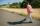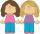# Chase

Paul travel at 60 km/h. After 18 km ride found that he forgot an important book. His brother Thomas behind him carrying it. How fast must Thomas go to catch up with Paul in 31 minutes?

Result

v2 =  94.8 km/h

#### Solution:Leave us a comment of example and its solution (i.e. if it is still somewhat unclear...):Be the first to comment!#### To solve this example are needed these knowledge from mathematics:

Do you have a linear equation or system of equations and looking for its solution? Or do you have quadratic equation? Do you want to convert length units?

## Next similar examples:

1. Cyclist 9A cyclist travels at a a speed of 4.25 km per hour. At that rate, how far can he travel in 3.75 hours?
2. Forest nurseryIn the forest nursery after winter, they found that 1/10 stems died out of them. For them, they land 193 new spruces. How many spruces are in the forest nursery?
3. Bus 14Boatesville is 65.35 kilometers from Stanton. A bus traveling from Stanton is 24.13 kilometers from Boatesville. How far has the bus traveled?
4. How oldThe student who asked how many years he answered: "After 10 years I will be twice as old than as I was four years ago. How old is student?
5. Unknown number 11That number increased by three equals three times itself?
6. Simple equationSolve for x: 3(x + 2) = x - 18
7. Average speedMichal went out of the house by car at speed 98 km/h. He came into the goal place for 270 minutes. Determine the distance between the two places.
8. Simple equationSolve the following simple equation: 2. (4x + 3) = 2-5. (1-x)
9. Dropped sheetsThree consecutive sheets dropped from the book. The sum of the numbers on the pages of the dropped sheets is 273. What number has the last page of the dropped sheets?
10. Find xSolve: if 2(x-1)=14, then x= (solve an equation with one unknown)
11. NormThree workers planted 3555 seedlings of tomatoes in one dey. First worked at the standard norm, the second planted 120 seedlings more and the third 135 seedlings more than the first worker. How many seedlings were standard norm?
12. PedestrianDana started at 10:00 from the point A to point B. These points are distant 12 km. Determine how fast Daniel went when the place B arrived at 11:54. Speed express in km/h.
13. Bed timeTiffany was 5 years old; her week night bedtime grew by ¼ hour each year. If, at age 18, her curfew time is 11pm, what was her bed time when she was 5 years old?
14. Equation 29Solve next equation: 2 ( 2x + 3 ) = 8 ( 1 - x) -5 ( x -2 )
15. Negative in equation2x + 3 + 7x = – 24, what is the value of x?
16. TimeageSeven times of my age is 8 less than the largest two-digit number. How old I am?
17. SistersSisters Hanka and Vera goes mostly tograndmother by bicycle. Journey took Hanka 30 minutes and Vera 20 minutes. How long will Vera catch up with Hanka when started from home five minutes later than Hanka?